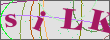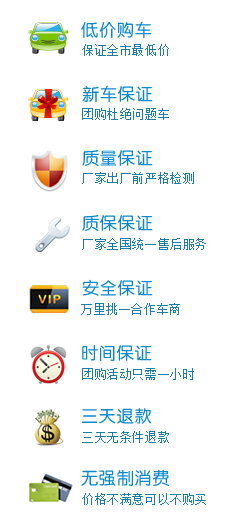# 活动中心

## [郑州] 乐活baby嘉年华 中原首届亲子赏车会

 团购时间：2015年07月31日 累计报名：5 人已报名 团购价格：现场公布 已报名：5 人 剩余时间：已结束 团购亮点：购车享优惠，更有豪礼相送

## [郑州] 广本智通 盛夏钜惠购车季

 团购时间：2015年07月31日 累计报名：15 人已报名 团购价格：现场公布 已报名：5 人 剩余时间：已结束 团购亮点：购车享优惠，更有豪礼相送

## [郑州] 广本智通快乐购车季

 团购时间：2015年06月30日 累计报名：18 人已报名 团购价格：现场公布 已报名：18 人 剩余时间：已结束 团购亮点：

## [郑州] 河南万盛总经理签售会，5月网络客户专享团购价

 团购时间：2015年05月31日 累计报名：26 人已报名 团购价格：现场公布 已报名：30 人 剩余时间：已结束 团购亮点：

## [郑州] 东风本田 全系舞动

 团购时间：2015年05月01日 累计报名：36 人已报名 团购价格：现场公布 已报名：13 人 剩余时间：已结束 团购亮点：在线订购，更有豪礼相送

## [郑州] 开春购车特惠季 广汽本田智通店

 团购时间：2015年04月30日 累计报名：36 人已报名 团购价格：现场公布 已报名：22 人 剩余时间：已结束 团购亮点：现金优惠最多、买车损险送三责险、港澳双飞游

## [郑州] 【创驰双雄 试不可挡】长安马自达试驾有礼活动火热开启

 团购时间：2014年12月31日 累计报名：56 人已报名 团购价格：活动现场公布 已报名：22 人 剩余时间：已结束 团购亮点：购车送好礼、更多现金补贴、更多油卡相送

## [郑州] 东风本田[一起来·放肆购]热门车系河南华林4S店火热开团

 团购时间：2014年11月17日 累计报名：107 人已报名 团购价格：活动现场公布 已报名：45 人 剩余时间：已结束 团购亮点：0利息、0抵押、担保、0折旧、千元补贴增值换购

## [郑州] 郑东天道全系开团 长安福特[翼]起享福 第二波火热来袭

 团购时间：2014年10月31日 累计报名：302 人已报名 团购价格：活动现场公布 已报名：268 人 剩余时间：已结束 团购亮点：免费参团、低价保证、4S店提车

## [郑州] 河南新裕隆全系大型团购会火热开启,快来报名!

 团购时间：2014年10月01日 累计报名：1368 人已报名 团购价格：团购现场公布 已报名：281 人 剩余时间：已结束 团购亮点：低价有好礼

### 团购活动流程

• 您可在报名页面填写报名信息，或拨打咨询电话报名，报名成功后等待客服预约。

• 4S店客服至少提前1天致电您，确认车型及到店时间等。

• 参与团购活动、了解优惠价格及相关政策。

• 您如果对价格政策满意，即可办理订车或购车相关手续。

• 了解您对本次团购活动的满意度建议及意见。

• 邀请您加入车行网车友会。

### 团购活动介绍

车行天下大型汽车团购活动，放心+低价+礼品！
【活动一】全系热卖车型提供充足现车! 【足量现车】
【活动二】所有到店团友都将获得精美礼品！【来就送】
【活动三】厂家特批，4S店优惠抢购政策，当期最低价【劲爆团购价】
【活动四】准时现场公开报价，保证透明公平，拒绝隐形消费！【公开价格】
【活动五】全程免费参与，车行天下团长现场解答，千万会员口碑保证！【安全放心】
【活动六】现场看车,全程讲解、试乘试驾，省时省钱省心！【仅限当天】
【活动七】活动时限内订车，保险、美容、装潢尽享【折上折】
【活动八】成为车行天下会员，购车有优惠，分享有礼品，用车上论坛【车友会】

### 我期待的团购车型

•### 车行天下购车保证### 更多>>精彩活动集锦

• 关注车行天下，掌握最新车市动态
•• 车行天下微信
﻿
• 快速找车
• 选择品牌
• 选择品牌
• A  奥迪
• A  阿斯顿·马丁
• A  阿尔法·罗密欧
• B  宝沃
• B  布加迪
• B  巴博斯
• B  保时捷
• B  宾利
• B  奔驰
• B  宝马
• B  本田
• B  别克
• B  标致
• B  比亚迪
• B  宝骏
• B  北汽制造
• B  北汽新能源
• B  北汽幻速
• B  北汽威旺
• B  北京汽车
• B  奔腾
• B  北汽绅宝
• B  北汽昌河
• C  长安欧尚
• C  长安
• C  长安凯程
• C  长城
• D  大众
• D  道奇
• D  DS
• D  东南
• D  东风风神
• D  东风风行
• D  东风小康
• D  东风风度
• D  东风
• F  福特
• F  丰田
• F  菲亚特
• F  法拉利
• F  福田
• F  福迪
• F  福汽启腾
• G  观致
• G  广汽传祺
• G  广汽吉奥
• G  GMC
• H  红旗
• H  汉腾汽车
• H  哈弗
• H  哈飞
• H  海格
• H  海马
• H  华颂
• H  黄海
• H  华泰
• H  恒天
• J  捷途
• J  几何汽车
• J  捷达
• J  吉利汽车
• J  捷豹
• J  Jeep
• J  江淮
• J  江铃
• J  金杯
• J  九龙
• J  金旅
• K  凯翼
• K  凯迪拉克
• K  克莱斯勒
• K  科尼塞克
• K  卡威
• K  开瑞
• L  路虎
• L  林肯
• L  劳斯莱斯
• L  兰博基尼
• L  雷克萨斯
• L  铃木
• L  领克
• L  雷诺
• L  理念
• L  力帆
• L  莲花汽车
• L  猎豹
• L  路特斯
• L  陆风
• M  马自达
• M  MG
• M  MINI
• M  玛莎拉蒂
• M  摩根
• M  迈凯轮
• N  纳智捷
• O  欧拉
• O  欧宝
• O  讴歌
• O  欧朗
• Q  奇瑞
• Q  起亚
• Q  启辰
• R  日产
• R  荣威
• R  瑞麒
• S  SERES赛力斯
• S  三菱
• S  斯威汽车
• S  萨博
• S  smart
• S  斯柯达
• S  斯巴鲁
• S  思铭
• S  双龙
• S  上汽大通
• S  双环
• T  特斯拉
• T  腾势
• W  蔚来
• W  沃尔沃
• W  WEY
• W  五菱汽车
• W  五十铃
• W  威兹曼
• W  威麟
• X  现代
• X  雪佛兰
• X  星途
• X  雪铁龙
• X  小鹏汽车
• X  西雅特
• Y  一汽
• Y  英菲尼迪
• Y  英致
• Y  依维柯
• Y  野马汽车
• Y  永源
• Z  众泰
• Z  中华
• Z  中兴
• Z  知豆
• 选择车系
• 选择车系
• 车型对比
• 选择品牌
• 选择品牌
• A  奥迪
• A  阿斯顿·马丁
• A  阿尔法·罗密欧
• B  宝沃
• B  布加迪
• B  巴博斯
• B  保时捷
• B  宾利
• B  奔驰
• B  宝马
• B  本田
• B  别克
• B  标致
• B  比亚迪
• B  宝骏
• B  北汽制造
• B  北汽新能源
• B  北汽幻速
• B  北汽威旺
• B  北京汽车
• B  奔腾
• B  北汽绅宝
• B  北汽昌河
• C  长安欧尚
• C  长安
• C  长安凯程
• C  长城
• D  大众
• D  道奇
• D  DS
• D  东南
• D  东风风神
• D  东风风行
• D  东风小康
• D  东风风度
• D  东风
• F  福特
• F  丰田
• F  菲亚特
• F  法拉利
• F  福田
• F  福迪
• F  福汽启腾
• G  观致
• G  广汽传祺
• G  广汽吉奥
• G  GMC
• H  红旗
• H  汉腾汽车
• H  哈弗
• H  哈飞
• H  海格
• H  海马
• H  华颂
• H  黄海
• H  华泰
• H  恒天
• J  捷途
• J  几何汽车
• J  捷达
• J  吉利汽车
• J  捷豹
• J  Jeep
• J  江淮
• J  江铃
• J  金杯
• J  九龙
• J  金旅
• K  凯翼
• K  凯迪拉克
• K  克莱斯勒
• K  科尼塞克
• K  卡威
• K  开瑞
• L  路虎
• L  林肯
• L  劳斯莱斯
• L  兰博基尼
• L  雷克萨斯
• L  铃木
• L  领克
• L  雷诺
• L  理念
• L  力帆
• L  莲花汽车
• L  猎豹
• L  路特斯
• L  陆风
• M  马自达
• M  MG
• M  MINI
• M  玛莎拉蒂
• M  摩根
• M  迈凯轮
• N  纳智捷
• O  欧拉
• O  欧宝
• O  讴歌
• O  欧朗
• Q  奇瑞
• Q  起亚
• Q  启辰
• R  日产
• R  荣威
• R  瑞麒
• S  SERES赛力斯
• S  三菱
• S  斯威汽车
• S  萨博
• S  smart
• S  斯柯达
• S  斯巴鲁
• S  思铭
• S  双龙
• S  上汽大通
• S  双环
• T  特斯拉
• T  腾势
• W  蔚来
• W  沃尔沃
• W  WEY
• W  五菱汽车
• W  五十铃
• W  威兹曼
• W  威麟
• X  现代
• X  雪佛兰
• X  星途
• X  雪铁龙
• X  小鹏汽车
• X  西雅特
• Y  一汽
• Y  英菲尼迪
• Y  英致
• Y  依维柯
• Y  野马汽车
• Y  永源
• Z  众泰
• Z  中华
• Z  中兴
• Z  知豆
• 选择车系
• 选择车系
• 选择车型
• 选择车型
• 意见反馈